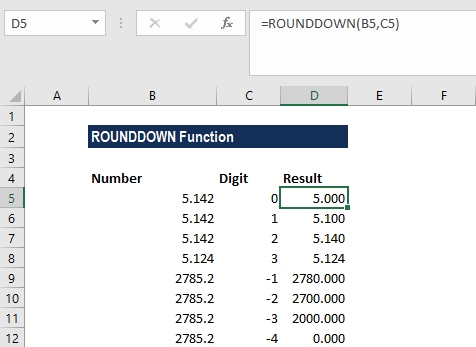# ROUNDDOWN Function

Rounds a given number down towards zero

## What is the ROUNDDOWN Function?

The ROUNDDOWN Function is categorized under Excel Math and Trigonometry functions. The function will round down towards zero. Basically, it does the opposite of the ROUNDUP function.

As a financial analyst, this function is useful as it helps round a number and eliminates the least significant digits, simplifying the notation but keeping close to the original value.

### Formula

=ROUNDDOWN(number,num_digits)

The ROUNDDOWN function uses the following arguments:

1. Number (required argument) – This is a real number that we wish to round down.
2. Num_digits (required argument) – This is the number of digits to which we want to round the number.

Now, if the num_digits argument is:

1. A positive value that is num_digits > number, it specifies the number of digits is rounded down to the specified number of decimal places.
2. Equal to zero, it specifies rounding to the nearest integer.
3. A negative value that is num_digits < number, it specifies the number of digits is rounded down to the specified number of decimal places.

### How to use the ROUNDDOWN Function in Excel?

To understand the uses of the ROUNDDOWN function, let’s consider an example:

#### Example

Suppose we are given the following data:

NumberDigitsRemarks
5.20Round down to the nearest whole number
51.421Round down to 1 decimal place
5.1422Round down to 2 decimal places
2785.23Round down to 3 decimal places
2785.2-1Round down to the nearest 10
2785.2-2Round down to the nearest 100
2785.2-3Round down to the nearest 1000
2785.2-4Round down to the nearest 10000

The results we get in Excel are shown below:### Things to remember

1. The ROUNDDOWN function behaves like the ROUND function, except that it always rounds a number down.
2. It can round either to the left or right of the decimal point.
3. Unlike standard rounding, where numbers less than 5 are rounded down, the function always rounds numbers 1-9 down.

Thanks for reading CFI’s guide to the Excel ROUNDDOWN function. By taking the time to learn and master these Excel functions, you’ll significantly speed up your financial analysis. To learn more, check out these additional CFI resources:

• Excel Functions for Finance